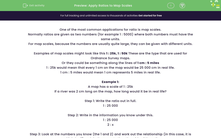# Apply Ratios to Map Scales

In this worksheet, students will practise applying ratios to map scales.Key stage:  KS 4

Year:  GCSE

GCSE Subjects:   Maths

GCSE Boards:   AQA, Eduqas, Pearson Edexcel, OCR,

Curriculum topic:   Geometry and Measures, Mensuration

Curriculum subtopic:   Mensuration and Calculation Units and Measurement

Difficulty level:#### Worksheet Overview

One of the most common applications for ratio is map scales.

Normally ratios are given as two numbers (for example 1 : 5000) where both numbers must have the same units.

For map scales, because the numbers are usually quite large, they can be given with different units.

Examples of map scales might look like this 1 : 25k, 1 : 50k These are the type that are used for Ordnance Survey maps.

Or they could be something along the lines of 1 cm : 5 miles

1 : 25k would mean that every 1 cm on the map would be 25 000 cm in real life.

1 cm : 5 miles would mean 1 cm represents 5 miles in real life.

Example 1:

A map has a scale of 1 : 25k

If a river was 2 cm long on the map, how long would it be in real life?

Step 1: Write the ratio out in full.

1 : 25 000

Step 2: Write in the information you know under this.

1 : 25 000

2 : x

Step 3: Look at the numbers you know (the 1 and 2) and work out the relationship (in this case, it is multiply by 2) and do the same to the other side of the ratio.

1 : 25 000

2 : 50 000

This means that the river is 50 000 cm long in real life.

Example 2:

Two car parks are 15 miles apart.

If the scale for a map is 1 cm : 5 miles, how far apart are the car parks on the map?

Step 1: Write the ratio out in full.

1 cm : 5 miles

Step 2: Write in the information you know under this.

1 cm : 5 miles

x  : 15 miles

Step 3: Look at the numbers you know (the 5 and 15) and work out the relationship (in this case, it is multiply by 3) and do the same to the other side of the ratio.

1 cm : 5 miles

3 cm : 15 miles

This means that the car parks are 3 cm apart on the map.

Time for some questions now.

### What is EdPlace?

We're your National Curriculum aligned online education content provider helping each child succeed in English, maths and science from year 1 to GCSE. With an EdPlace account you’ll be able to track and measure progress, helping each child achieve their best. We build confidence and attainment by personalising each child’s learning at a level that suits them.

Get started